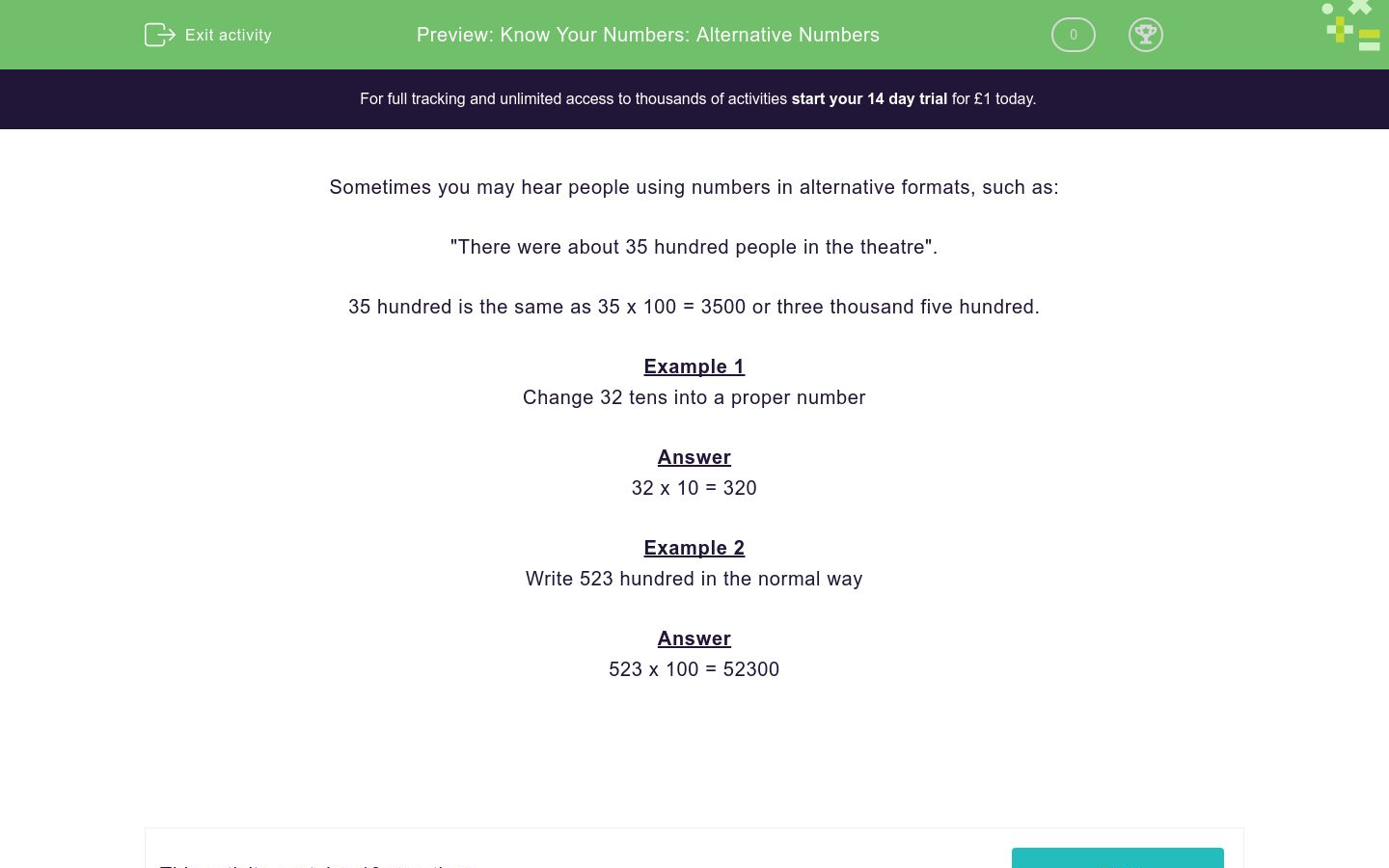# Know Your Numbers: Alternative Numbers

In this worksheet, students write numbers given in alternative formats in a more standard way.Key stage:  KS 2

Curriculum topic:   Number: Number and Place Value

Curriculum subtopic:   Know Numbers to 1 000 000

Difficulty level:### QUESTION 1 of 10

Sometimes you may hear people using numbers in alternative formats, such as:

"There were about 35 hundred people in the theatre".

35 hundred is the same as 35 x 100 = 3500 or three thousand five hundred.

Example 1

Change 32 tens into a proper number

32 x 10 = 320

Example 2

Write 523 hundred in the normal way

523 x 100 = 52300

Change the following number into a proper number:

35 hundred

Change the following number into a proper number:

43 hundred

Change the following number into a proper number:

43 tens

Change the following number into a proper number:

413 tens

Change the following number into a proper number:

40 tens

Change the following number into a proper number:

40 hundreds

Change the following number into a proper number:

400 hundreds

Change the following number into a proper number:

4000 hundreds

Change the following number into a proper number:

4200 tens

Change the following number into a proper number:

42000 tens

• Question 1

Change the following number into a proper number:

35 hundred

3500
EDDIE SAYS
35 x 100 = 3500
• Question 2

Change the following number into a proper number:

43 hundred

4300
EDDIE SAYS
43 x 100 = 4300
• Question 3

Change the following number into a proper number:

43 tens

430
EDDIE SAYS
43 x 10 = 430
• Question 4

Change the following number into a proper number:

413 tens

4130
EDDIE SAYS
413 x 10 = 4130
• Question 5

Change the following number into a proper number:

40 tens

400
EDDIE SAYS
40 x 10 = 400
• Question 6

Change the following number into a proper number:

40 hundreds

4000
EDDIE SAYS
40 x 100 = 4000
• Question 7

Change the following number into a proper number:

400 hundreds

40000
EDDIE SAYS
400 x 100 = 40000
• Question 8

Change the following number into a proper number:

4000 hundreds

400000
EDDIE SAYS
4000 x 100 = 400000
• Question 9

Change the following number into a proper number:

4200 tens

42000
EDDIE SAYS
4200 x 10 = 42000
• Question 10

Change the following number into a proper number:

42000 tens

420000
EDDIE SAYS
42000 x 10 = 420000
---- OR ----

Sign up for a £1 trial so you can track and measure your child's progress on this activity.

### What is EdPlace?

We're your National Curriculum aligned online education content provider helping each child succeed in English, maths and science from year 1 to GCSE. With an EdPlace account you’ll be able to track and measure progress, helping each child achieve their best. We build confidence and attainment by personalising each child’s learning at a level that suits them.

Get started